# Percentages + sets - math problems

#### Number of problems found: 12

• TVsProduction of television sets increased from 3,500 units to 4,200 units. Calculate the percentage of production increase.
• AceFrom complete sets of playing cards (32 cards), we pulled out one card. What is the probability of pulling the ace?
• Socks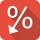One pair of socks worth CZK 27. Set of 3 pairs of these socks are sold with 10% discount. How many will we pay CZK for two offered sets of socks?
• Left handedIt is known that 25% of the population is left-handed. What is the probability that there is a maximum of three left-handers at a seminar where there are 30 participants?
• Here isHere is a data set (n=117) that has been sorted. 10.4 12.2 14.3 15.3 17.1 17.8 18 18.6 19.1 19.9 19.9 20.3 20.6 20.7 20.7 21.2 21.3 22 22.1 22.3 22.8 23 23 23.1 23.5 24.1 24.1 24.4 24.5 24.8 24.9 25.4 25.4 25.5 25.7 25.9 26 26.1 26.2 26.7 26.8 27.5 27.6 2
• Alarm systems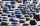What is the probability that at least one alarm system will signal the theft of a motor vehicle when the efficiency of the first system is 90% and of the independent second system 80%?
• Families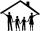In class we investigated how many children have family in which its live. How many% of pupils live in families with more than two children? If five families each have one child in 9 families have 2 children, in 5 families have 3 children, in 2 families ha
• Hazard game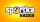In the Sportka hazard game, 6 numbers out of 49 are drawn. What is the probability that we will win: a) second prize (we guess 5 numbers correctly) b) the third prize (we guess 4 numbers correctly)?
• Two doctorsDoctor A will determine the correct diagnosis with a probability of 93% and doctor B with a probability of 79%. Calculate the probability of proper diagnosis if both doctors diagnose the patient.
• Summer camp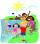Out of the 180 students at a summer camp, 72 signed up for canoeing. Twenty-three students signed up for trekking, and 13 of those students also signed up for canoeing. Use a two-way table to organize the information and answer the following question: App
• Utopia IslandA probability of disease A on the island of Utopia is 40%. A probability of occurrence among the men of this island, which make up 60% of all the population (the rest are women), is 50%. What is the probability of occurrence of A disease among women on Ut
• The universityAt a certain university, 25% of students are in the business faculty. Of the students in the business faculty, 66% are males. However, only 52% of all students at the university are male. a. What is the probability that a student selected at random in the

We apologize, but in this category are not a lot of examples.
Do you have an interesting mathematical word problem that you can't solve it? Submit a math problem, and we can try to solve it.

We will send a solution to your e-mail address. Solved examples are also published here. Please enter the e-mail correctly and check whether you don't have a full mailbox.

Please do not submit problems from current active competitions such as Mathematical Olympiad, correspondence seminars etc...

Our percentage calculator will help you quickly calculate various typical tasks with percentages. Percentages Problems. Sets - math word problems.